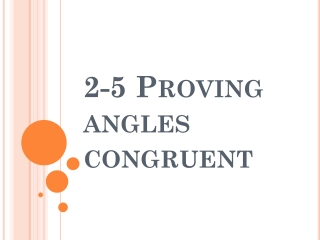DownloadDownload Presentation2-5 Proving angles congruent

# 2-5 Proving angles congruent

Download Presentation## 2-5 Proving angles congruent

- - - - - - - - - - - - - - - - - - - - - - - - - - - E N D - - - - - - - - - - - - - - - - - - - - - - - - - - -
##### Presentation Transcript

1. 2-5 Proving angles congruent

2. Vertical angles • Two angles whose sides are opposite rays 1 4 3 2

3. Adjacent angles • Two coplanar angles with a common side, a common vertex, and no common interior points 3 4 1 2

4. Complementary angles • Two angles that ADD up to 90˚ • Each angle is called the complement of the other 50° 1 A 2 40° B

5. Supplementary Angles • Two angles that ADD up to 180° • Each angle is called the supplement of the other 105° 75° 3 4

6. Examples! • 1. Identify Angle Pairs • Complementary • Supplementary • Vertical • Adjacent 2 1 3 5 4

7. 2. What can you tell from the picture? • About ∠1 and ∠2? • What type of angles are ∠2 and ∠3? • What type of angles are ∠4 and ∠5? • What type of angles are ∠1 and ∠4? 3 2 4 1 5

8. Vertical Angles Theorem (2-1) • Vertical angles are congruent (≅) • ∠1 ≅ ∠2 and ∠3 ≅ ∠4 1 4 3 2

9. Using the vertical angles theorem • Find the value of x. • Find the value of each angle. 4x (3x + 35)

10. Congruent Supplements Theorem (2-2) • If two angles are supplements of the same angle (or of congruent angles), then the two angles are congruent. • ∠1 and ∠2 are supplementary (∠2 is a supplement of ∠1) • ∠1 and ∠3 are supplementary (∠3 is a supplement of ∠1) • So ∠2 ≅ ∠3 2 3 1

11. Congruent Complements Theorem (2-3) • If two angles are complements of the same angle (or of congruent angles), then the two angles are congruent. • Theorem 2-4: All right angles are congruent. • Theorem 2-5: If two angles are congruent and supplementary, then each is a right angle.

12. Ticket Out the door